# Random Forests in Machine Learning

## Random Forests in Machine Learning

In this article, I am going to discuss Random Forests in Machine Learning with Examples. Please read our previous article where we discussed Model Evaluation for Classification in Machine Learning with Examples.

##### Random Forests in Machine Learning

Random Forests produce a large number of categorization trees. Put the input vector down each of the trees in the forest to categorize a new object from an input vector. Each tree assigns a categorization, and we refer to this as the tree’s “vote.” The classification with the highest votes is chosen by the forest (over all the trees in the forest).

The following stages will help us understand how the Random Forest algorithm works.

1. Step1: Begin by selecting random samples from a dataset.
2. Step2: For each sample, this algorithm will create a decision tree. The forecast result from each decision tree will then be obtained.
3. Step3: Voting will be done for each expected outcome in this step.
4. Step4: Finally, choose the prediction result with the most votes as the final forecast result.
##### The Random Forest method has the following advantages –
1. By averaging or integrating the outputs of different decision trees, it addresses the problem of overfitting.
2. Random forests perform better than a single decision tree for a wide range of data items.
3. Even when a major amount of the data is missing, the Random Forest algorithms maintain high accuracy.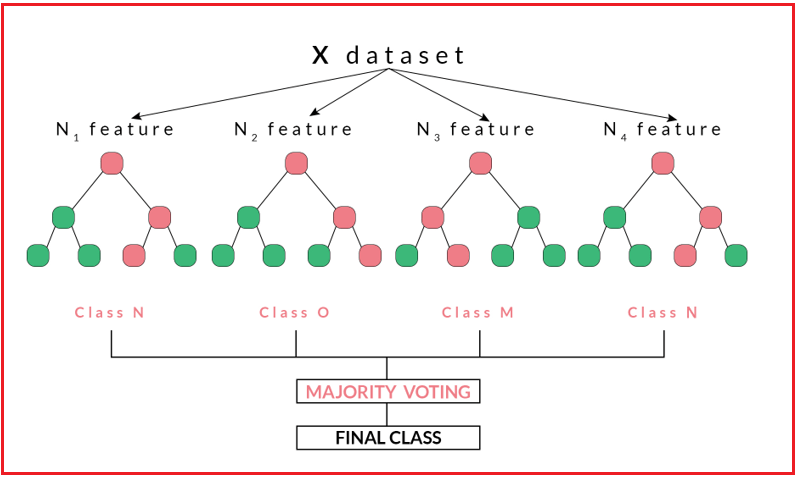##### Features of Random Forest in Machine Learning

Following are the major features of the Random Forest Algorithm –

1. It is the most accurate algorithm currently available.
2. It works well with huge databases.
3. It can handle tens of thousands of input variables without deleting any of them.
4. It calculates the importance of several variables in the classification.
5. As the forest grows, it generates an internal unbiased estimate of the generalization error.
6. It offers a good strategy for guessing missing data and keeps its accuracy even when a high percentage of the data is missing.
7. It includes methods for balancing inaccuracy in uneven data sets in class populations.
8. The forests that are created can be preserved and used on other data in the future.
9. Prototypes are created that show the relationship between the variables and the classification.
10. It calculates distances between pairs of examples, which can be useful in clustering, detecting outliers, or giving fascinating views of the data (by scale).
11. Unlabeled data can be used to create unsupervised clustering, data visualizations, and outlier identification using the capabilities described above.
12. It provides a mechanism for finding variable interactions through experimentation.
##### Variable Importance

In many (commercial) circumstances, having both an accurate and interpretable model is equally vital. We often question why our model’s house price prediction is so high/low and which features are most essential in determining the forecast, in addition to what our model’s house price prediction is. Another example is forecasting customer turnover – it’s great to have a model that can accurately predict which customers are likely to quit, but figuring out which variables are crucial might aid in early detection and possibly even product/service improvement!

Knowing the value of features as determined by machine learning models can help you in a variety of ways, including:

1. By better understanding the model’s logic, you may not only ensure that it is right but also work to improve it by focusing exclusively on the most relevant variables.
2. The above can be used to pick variables – you can eliminate x variables that aren’t that important and achieve identical or better results in a fraction of the time.
3. In some commercial situations, sacrificing some accuracy for interpretability makes sense. When a bank rejects a loan application, for example, it must have a rationale for doing so that can be communicated to the customer.

When we train a Random Forest model on a Data Set with specific characteristics, the model object we get can inform us which features were the most relevant during training, i.e. which ones had the most influence on the target variable.

This variable importance is determined for each tree in a forest with many distinct trees and then averaged across the forest to generate a single metric per feature.

We can use this measure to rank the features by relevance and retrain our random forest model with only those features, thus performing a feature selection step and rejecting the rest.

##### Let’s take an example of the preprocessed titanic dataset –
```# First we build and train our Random Forest Model
from sklearn.ensemble import RandomForestClassifier
from sklearn.metrics import accuracy_score
rf = RandomForestClassifier(random_state=42)
rf.fit(x_train, y_train)
y_pred = rf.predict(x_test)
print(accuracy_score(y_test, y_pred))
```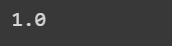```feature_scores = pd.Series(rf.feature_importances_, index=x_train.columns).sort_values(ascending=False)
feature_scores
```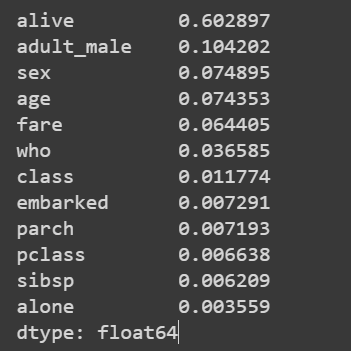### Case Study in Healthcare

#### Introduction

##### Coronary Artery Disease:
1. It refers to the narrowing or blockage of the coronary arteries, usually caused by the build-up of cholesterol and fatty deposits (called plaques) on the inner walls of the arteries.
2. These plaques can restrict blood flow to the heart muscle by physically clogging the artery or by causing abnormal artery tone and function.
3. This can cause chest pain called Angina. When one or more of the coronary arteries are completely blocked, a heart attack may occur.

There are various factors that affect coronary heart disease. These are as follows:

1. Risk factors like gender, family history, race, ethnicity, etc. are non-modifiable (unable to cure).
2. Risk factors like cigarette smoking, high blood cholesterol levels, high blood pressure, physical inactivity, etc. are modifiable.
##### Problem Statement
1. Heart Trek, an American hospital, specializes in the identification and treatment of heart-related diseases.
2. They do a variety of tests to identify the level of risk a patient might be in.
3. After detecting the level of risk, they perform treatment which could get late if a patient is at high risk.
4. These tests consume a lot of time, and meanwhile, if a patient is at high risk, it could lead to the death of the patient.
5. Over the past years they have accumulated data regarding history of patients in addition to detailed information.
6. They require an automated solution which could prevent these time-consuming tests.
7. So, they hired a team of data scientists to find patterns out of the data which could prevent high latency of events.
8. The target feature in this dataset is named as the target which we need to predict.
##### Importing Libraries
```# For Panel Data Analysis
import pandas as pd
from pandas_profiling import ProfileReport
import pandas.util.testing as tm
pd.set_option('display.max_columns', None)
pd.set_option('display.max_colwidth', None)
pd.set_option('display.max_rows', None)
pd.set_option('mode.chained_assignment', None)

# For Numerical Python
import numpy as np

# For Random seed values
from random import randint

# For Scientific Python
from scipy import stats

# For datetime
from datetime import datetime as dt

# For Data Visualization
import matplotlib.pyplot as plt
%matplotlib inline
import seaborn as sns

# For Preprocessing
from sklearn.preprocessing import StandardScaler

# For Feature Selection
from sklearn.feature_selection import SelectFromModel

# For Feature Importances
from yellowbrick.model_selection import FeatureImportances

# For metrics evaluation
from sklearn.metrics import precision_recall_curve, classification_report, plot_confusion_matrix

# For Data Modeling
from sklearn.model_selection import train_test_split, cross_val_score, GridSearchCV
from sklearn.linear_model import LogisticRegression
from sklearn.ensemble import RandomForestClassifier

# To Disable Warnings
import warnings
warnings.filterwarnings(action = "ignore")

print("Data Shape: ",data.shape)
```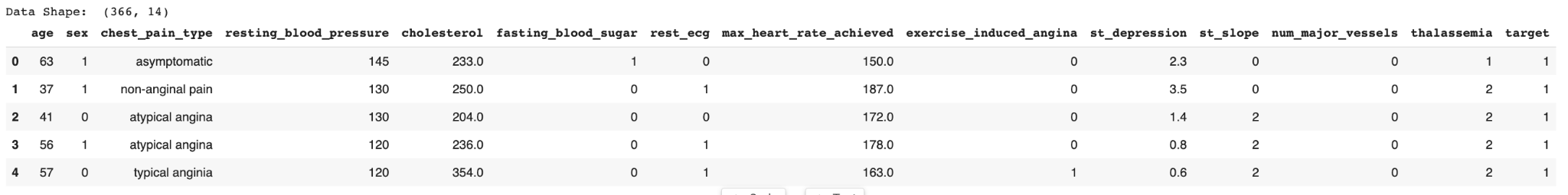###### Data Description
```print('Described Column Length:', len(data.describe().columns))
data.describe()
```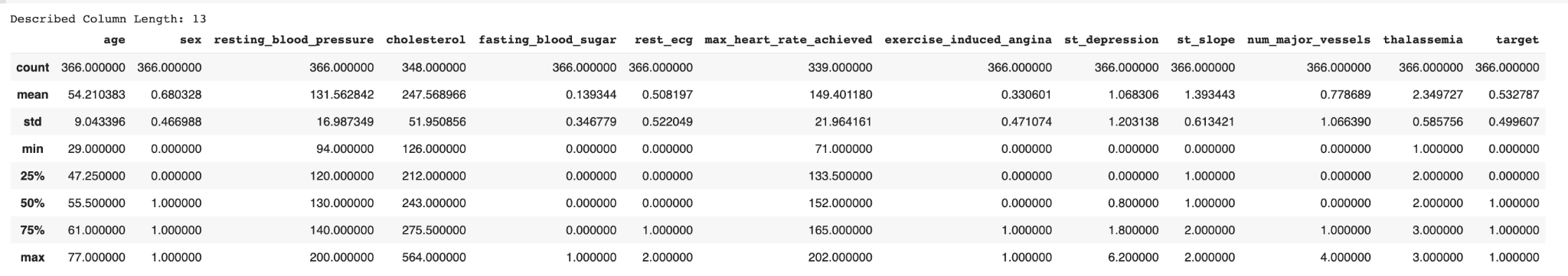data.info()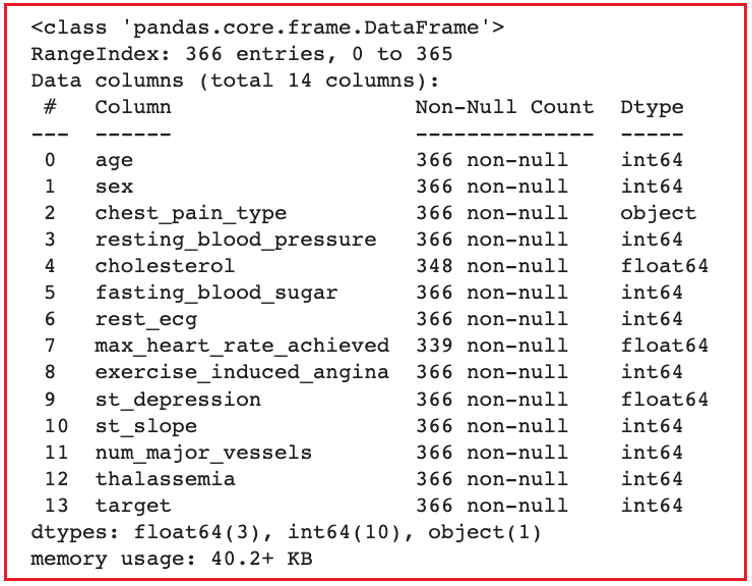##### Data Pre-Processing
```data['cholesterol'] = data['cholesterol'].replace(np.nan, data['cholesterol'].median())
data['max_heart_rate_achieved'] = data['max_heart_rate_achieved'].replace(np.nan, data['max_heart_rate_achieved'].median())

print('Contains Redundant Records?:', data.duplicated().any())
print('Duplicate Count:', data.duplicated().sum())
```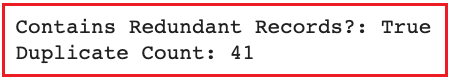```before_shape = data.shape
print('Data Shape [Before]:', before_shape)

data.drop_duplicates(inplace = True)

after_shape = data.shape
print('Data Shape [After]:', after_shape)

drop_nums = before_shape - after_shape
drop_percent = np.round(drop_nums / before_shape, decimals = 2) * 100

print('Drop Ratio:', drop_percent, '%')
```data[‘chest_pain_type’].unique()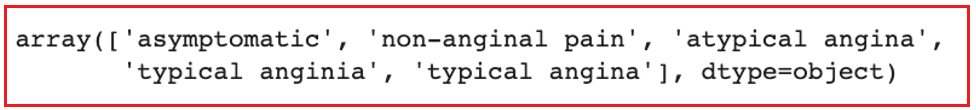data[‘chest_pain_type’] = data[‘chest_pain_type’].str.replace(pat = ‘typical angina’, repl = ‘typical angina’)

#### EDA

##### Question 1: What is the proportion of males and females having heart disease or not?
```males_data = data[data['sex'] == 1]
females_data = data[data['sex'] == 0]

figure = plt.figure(figsize = [12, 10])
plt.subplot(1, 2, 1)
space = np.ones(2)/10
males_data['target'].value_counts().plot(kind = 'pie', explode = space, fontsize = 14, autopct = '%3.1f%%', wedgeprops = dict(width = 0.15),
shadow = True, startangle = 160, figsize = [13.66, 7.68], legend = True, labels = ['', ''])
plt.legend(['No Heart Disease', 'Heart Disease'])
plt.ylabel('Males', size = 14)

plt.subplot(1, 2, 2)
space = np.ones(2)/10
females_data['target'].value_counts().plot(kind = 'pie', explode = space, fontsize = 14, autopct = '%3.1f%%', wedgeprops = dict(width=0.15),
shadow = True, startangle = 160, figsize = [13.66, 7.68], legend = True, labels = ['', ''])
plt.legend(['No Heart Disease', 'Heart Disease'])
plt.ylabel('Females', size = 14)
plt.suptitle('Proportion of Males and Females vs Heart Disease', size = 16)
plt.show()
```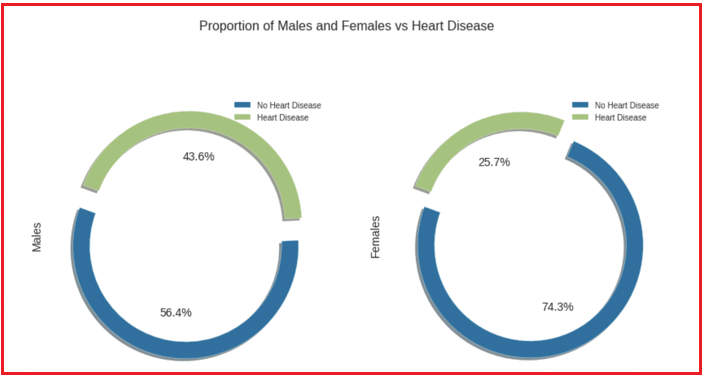##### Question 2: What is the proportion of males and females having different types of chest pain?
1. Typical Angina: It is the presence of substernal chest pain or discomfort that was provoked by exertion or emotional stress and was relieved by rest and/or nitroglycerin.
2. Non-Anginal Pain: It is defended with the possibility of avoiding diagnoses such as “atypical chest pain” or “atypical angina.”
3. Atypical Angina: It implies that the complaint is actually angina pecto- ris, though not conforming in every way to the expected or classic description.
4. Asymptomatic: It means neither causing nor exhibiting symptoms of the disease.
```males_data = data[data['sex'] == 1]
females_data = data[data['sex'] == 0]

figure = plt.figure(figsize = [12, 10])
plt.subplot(1, 2, 1)
space = np.ones(4)/10
males_data['chest_pain_type'].value_counts().plot(kind = 'pie', fontsize = 14, autopct = '%3.1f%%', wedgeprops = dict(width = 0.15),
shadow = True, startangle = 160, figsize = [13.66, 7.68], legend = True, labels = ['', '', '', ''])
plt.legend(['Typical Angina', 'Non-Anginal Pain', 'Atypical Angina', 'Asymptomatic'])
plt.ylabel('Males', size = 14)

plt.subplot(1, 2, 2)
space = np.ones(4)/10
females_data['chest_pain_type'].value_counts().plot(kind = 'pie', fontsize = 14, autopct = '%3.1f%%', wedgeprops = dict(width=0.15),
shadow = True, startangle = 160, figsize = [13.66, 7.68], legend = True, labels = ['', '', '', ''])
plt.legend(['Typical Angina', 'Non-Anginal Pain', 'Atypical Angina', 'Asymptomatic'])
plt.ylabel('Females', size = 14)
plt.suptitle('Males/Females vs Chest Pain', size = 16)
plt.show()
```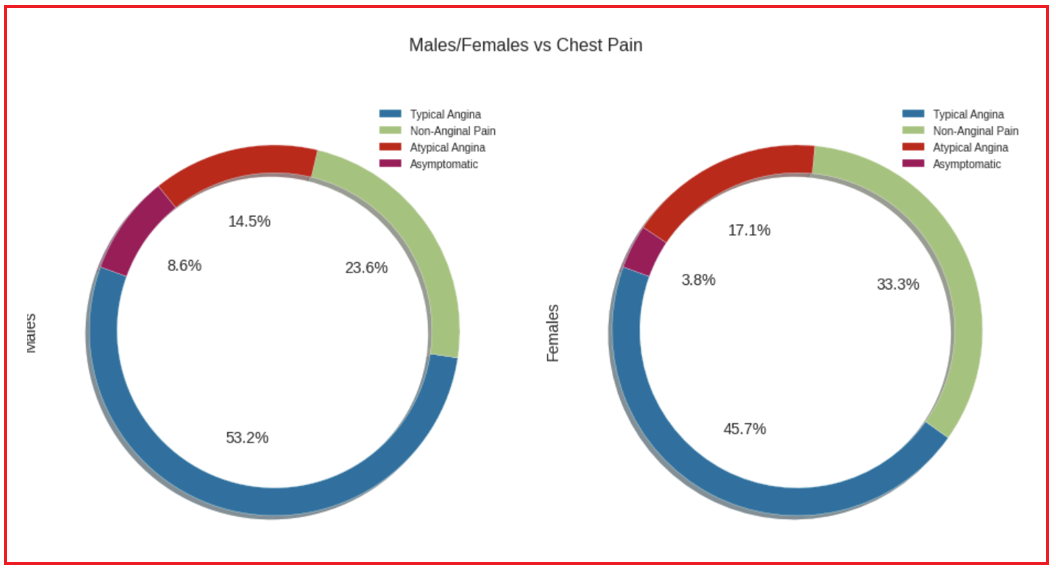##### Question 3: How much proportion of people have heart disease or not with respect to their fasting blood sugar?
```figure = plt.figure(figsize = [12, 7])

ax = sns.countplot(x = 'fasting_blood_sugar', hue = 'target', data = data, palette = ['#56DB7F', '#DB5E56'])
total = data.shape

for p in ax.patches:
percentage = '{:.2f}%'.format(100 * p.get_height() / total)
x = p.get_x() + p.get_width() / 3
y = p.get_y() + p.get_height()
ax.annotate(percentage, (x, y))

plt.xlabel('Fasting Blood Sugar', size = 14)
plt.xticks(ticks = [0, 1, 2], labels = ['Less than 120 mg/ml', 'Greater than 120 mg/ml'], rotation = 0)
plt.ylabel('Frequency', size = 14)
plt.legend(labels = ['No Heart Disease', 'Heart Disease'])
plt.title('Heart Disease vs Fasting Blood Sugar', size = 16)
plt.show()
```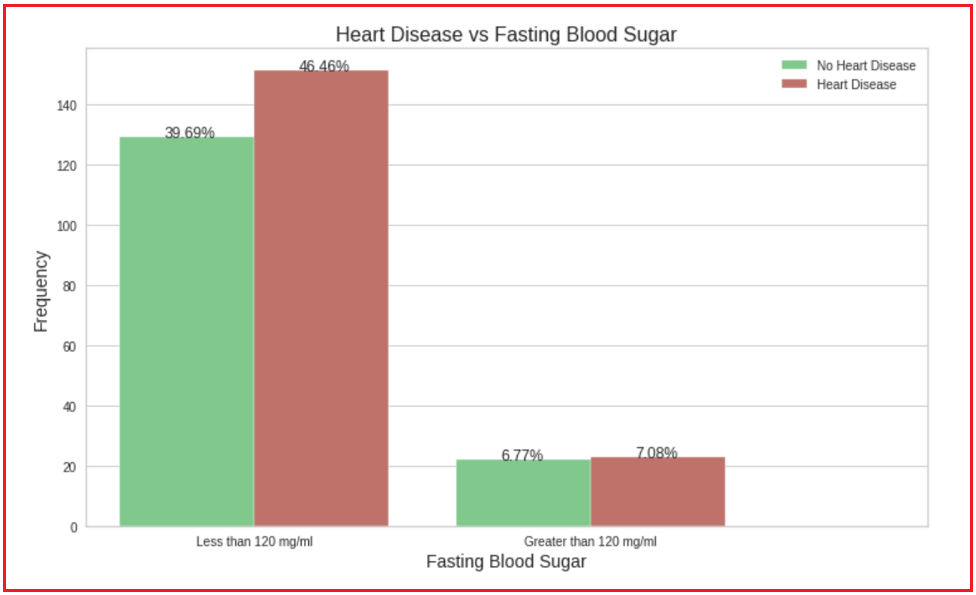##### Dummification
```data = pd.get_dummies(data = data, columns = ['chest_pain_type'])

X_train, X_test, y_train, y_test = train_test_split(X, y, test_size = 0.2, random_state = 42, stratify = y)

print('Training Data Shape:', X_train.shape, y_train.shape)
print('Testing Data Shape:', X_test.shape, y_test.shape)
```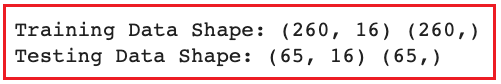#### Model Building

##### Logistic Regression
```log  = LogisticRegression(random_state = 42)
log.fit(X_train, y_train)

y_train_pred_count = log.predict(X_train)
y_test_pred_count = log.predict(X_test)

y_train_pred_proba = log.predict_proba(X_train)
y_test_pred_proba = log.predict_proba(X_test)

fig, (ax1, ax2) = plt.subplots(nrows = 1, ncols = 2, sharex = False, figsize=(15, 7))
plot_confusion_matrix(estimator = log, X = X_train, y_true = y_train, values_format = '.5g', cmap = 'YlGnBu', ax = ax1)
plot_confusion_matrix(estimator = log, X = X_test, y_true = y_test, values_format = '.5g', cmap = 'YlGnBu', ax = ax2)
ax1.set_title(label = 'Train Data', size = 14)
ax2.set_title(label = 'Test Data', size = 14)
ax1.grid(b = False)
ax2.grid(b = False)
plt.suptitle(t = 'Confusion Matrix', size = 16)
plt.show()
```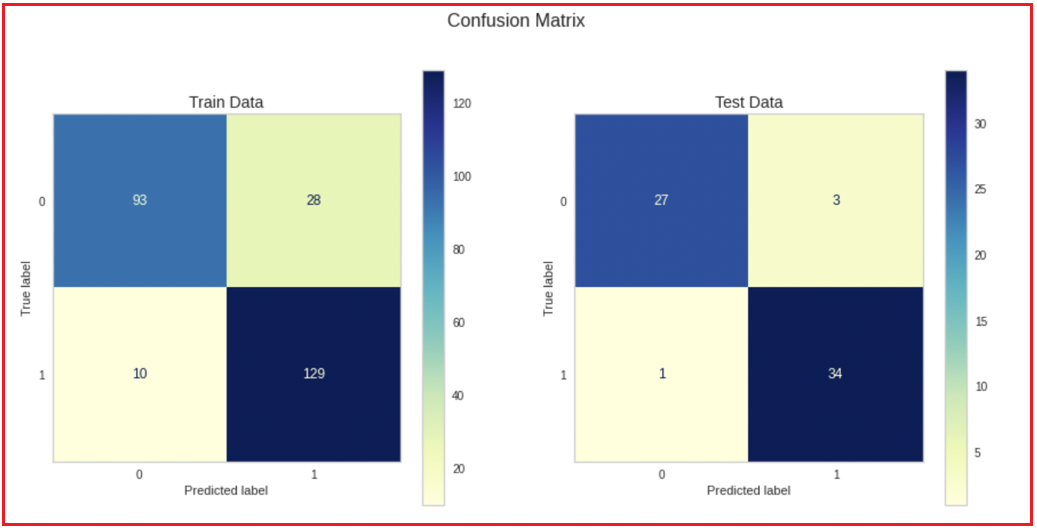```logistic_report_train = classification_report(y_train, y_train_pred_count)
logistic_report_test = classification_report(y_test, y_test_pred_count)
print('                    Training Report          ')
print(logistic_report_train)
print('                    Testing Report           ')
print(logistic_report_test)
```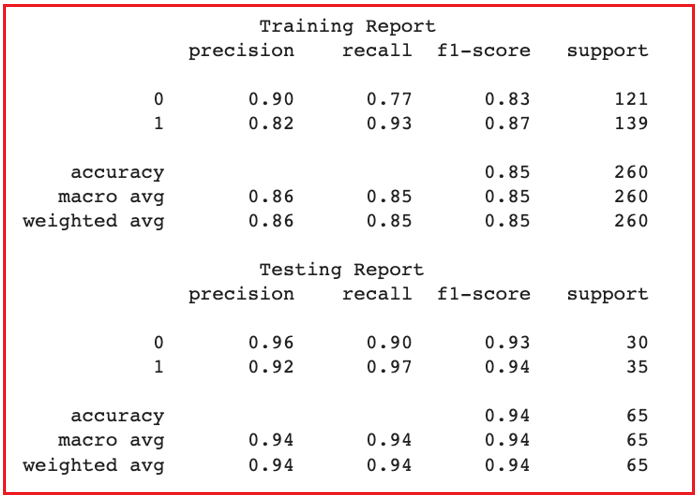##### Random Forest Classifier
```rfc = RandomForestClassifier(random_state = 42)
rfc.fit(X_train, y_train)

y_train_pred_count = rfc.predict(X_train)
y_test_pred_count = rfc.predict(X_test)

y_train_pred_proba = rfc.predict_proba(X_train)
y_test_pred_proba = rfc.predict_proba(X_test)

fig, (ax1, ax2) = plt.subplots(nrows = 1, ncols = 2, sharex = False, figsize=(15, 7))
plot_confusion_matrix(estimator = rfc, X = X_train, y_true = y_train, values_format = '.5g', cmap = 'YlGnBu', ax = ax1)
plot_confusion_matrix(estimator = rfc, X = X_test, y_true = y_test, values_format = '.5g', cmap = 'YlGnBu', ax = ax2)
ax1.set_title(label = 'Train Data', size = 14)
ax2.set_title(label = 'Test Data', size = 14)
ax1.grid(b = False)
ax2.grid(b = False)
plt.suptitle(t = 'Confusion Matrix', size = 16)
plt.show()
```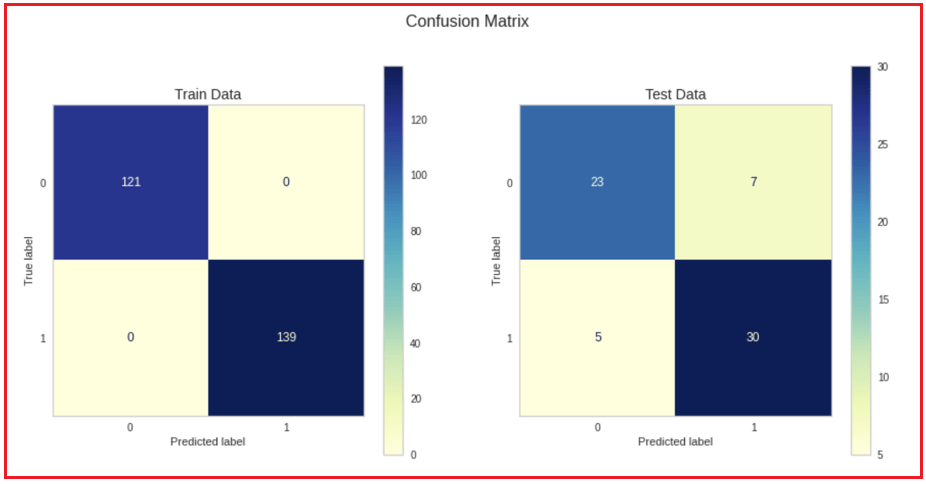```rfc_report_train = classification_report(y_train, y_train_pred_count)
rfc_report_test = classification_report(y_test, y_test_pred_count)
print('                    Training Report          ')
print(rfc_report_train)
print('                    Testing Report           ')
print(rfc_report_test)
```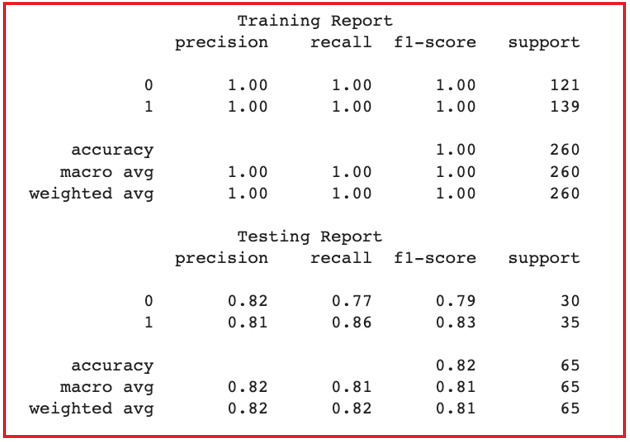In the next article, I am going to discuss Machine Learning Advanced Concepts with Examples. Here, in this article, I try to explain Random Forests in Machine Learning with Examples. I hope you enjoy this Random Forests in Machine Learning with Examples article.